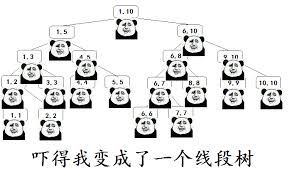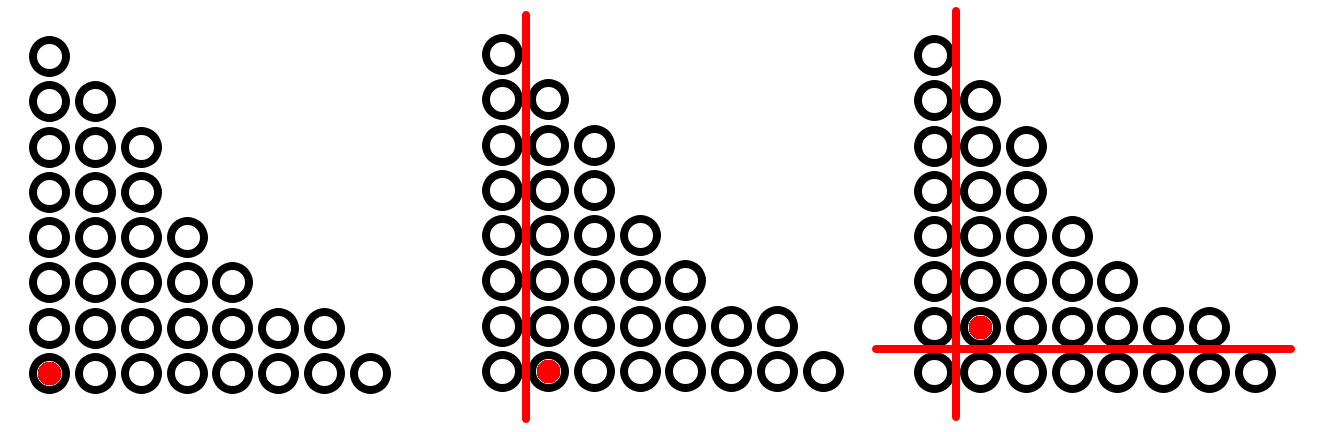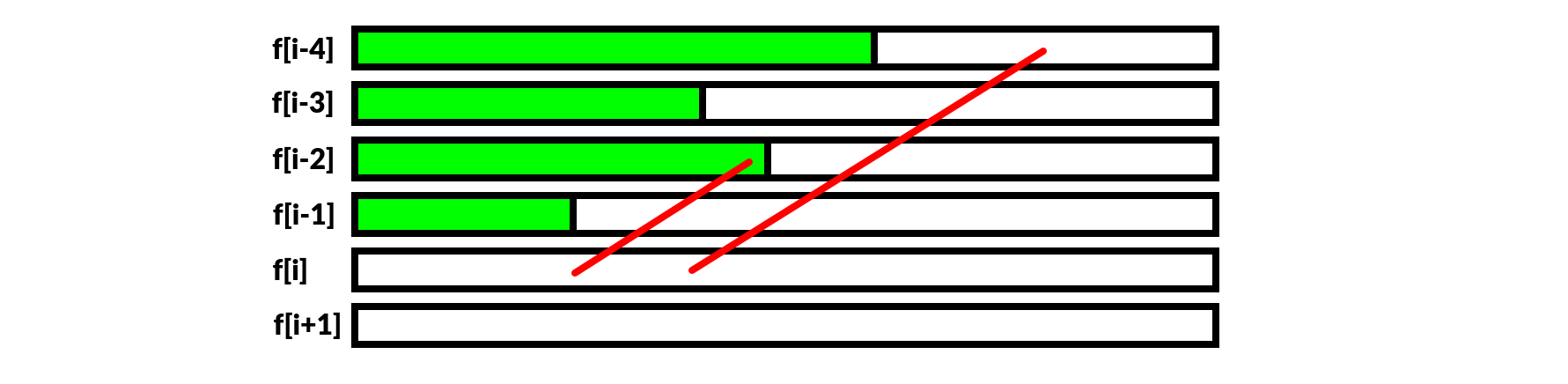2019.6.15 编入精选文章。

dls 讲博弈论：“你掉线，我随意”# 博弈的分类## 有向图游戏# DAGame 的判局

## DAGame 的和

DAGame 的某个局面 必胜，当且仅当 .

DAGame 的某个局面 必败，当且仅当 .

# SG 函数博弈

SG 博弈是 OI 中最常见的博弈类题目。其题目往往千奇百怪，但总的方法大概是：SG 函数是用于 ICG 游戏的判局，因此默认不能行动者判负。所以题面中不再说明胜负条件。除非条件有变

## 小练习

⽐如 .

### Exercise 3

``````#include<bits/stdc++.h>
#include<bitset>
using namespace std;

int SG;
bool vis;
int SG(int k){
if(vis[k])return SG[k];
vis[k]=1;
if(k==1)return SG[k]=0;
bool bit={0};
for(int i=1;i<k;i++)if(k%i==0)bit[SG(k-i)]=1;
for(int i=0;i<1000;i++)if(!bit[i])return SG[k]=i;
}
int main(){
for(int i=1;i<=20;i++)cout<<SG(i)<<' ';
return 0;
}
``````

### Exercise 4

``````#include<bits/stdc++.h>
#include<bitset>
using namespace std;
int gcd(int a,int b){return b?gcd(b,a%b):a;}
int SG;
bool vis;
int SG(int k){
if(vis[k])return SG[k];
vis[k]=1;
bool bit={0};
for(int i=1;i<=k;i++)if(gcd(i,k)==1)bit[SG(i)]=1;
for(int i=0;i<1000;i++)if(!bit[i])return SG[k]=i;
}
int main(){
for(int i=1;i<=20;i++)cout<<SG(i)<<' ';
return 0;
}
``````

## PE306

``````#include<bits/stdc++.h>
#include<bitset>
using namespace std;

int gcd(int a,int b){return b?gcd(b,a%b):a;}
int SG;
bool vis;
int SG(int k){
if(k==1)return 0;
if(k==2)return 1;
if(vis[k])return SG[k];
vis[k]=1;
bool bit={0};
for(int i=1;i+2<=k;i++)bit[SG(i)^SG(k-i-2)]=1;
for(int i=0;i<1000;i++)if(!bit[i])return SG[k]=i;
}
int main(){
for(int i=1;i<=1000;i++)cout<<' '<<SG(i);
return 0;
}
``````

## [POJ2311]Cutting Game

``````#include<cstdio>
#include<cstring>
#include<bitset>
using namespace std;
const int N=505;
int n,m,c[N][N];
int SG(int a,int b){
if(~c[a][b])return c[a][b];
bool bit[N]={0};
for(int i=2;i<=a-i;i++)bit[SG(i,b)^SG(a-i,b)]=1;
for(int i=2;i<=b-i;i++)bit[SG(a,i)^SG(a,b-i)]=1;
for(int i=0;i<N;i++)if(!bit[i])return c[a][b]=i;
}
int main(){
memset(c,-1,sizeof(c));
while(~scanf("%d%d",&n,&m))puts(SG(n,m)?"WIN":"LOSE");
return 0;
}
``````

## [LG1290] 欧几里德的游戏

，则

，则

``````#include<cstdio>
using namespace std;
typedef long long ll;
ll t,m,n;
bool SG(ll m,ll n){//m<n
if(!m)return 0;// 面对 m=0 的局面为负
if(n-m<m)return !SG(n%m,m);// 如果 SG!=0，则 SG(n,n%m +m)=0
else return 1;// 不管 SG(n,n%m) 的值，之后都不为 0
}
int main(){
scanf("%lld",&t);
while(t--){
scanf("%lld%lld",&m,&n);
if(m>n)m^=n^=m^=n;
puts(SG(m,n)?"Stan wins":"Ollie wins");
}
return 0;
}
``````

## [SDOI2009] E&D

``````const int N=100;
int SG[N][N];
bool vis[N][N];
int mex(bitset<N> bit){
int res=0;
while(bit[res])res++;
return res;
}
int SG(int a,int b){
if(a==1&&b==1)return 0;
if(vis[a][b])return SG[a][b];
bitset<N> bit;
for(int i=1;i<a;i++)bit[SG(i,a-i)]=1;
for(int i=1;i<b;i++)bit[SG(i,b-i)]=1;
return mex(bit);
}
``````

``````a=1    :00000000000
a=2    :00000000001
a=3    :00000000010
a=4    :00000000011
a=5    :00000000100
a=6    :00000000101
a=7    :00000000110
a=8    :00000000111
a=9    :00000001000
a=10    :00000001001
``````

``````#include<cstdio>
#include<bitset>
using namespace std;
int t,n;
int mex(int x){
int res=0;
while(x&1)x>>=1,res++;
return res;
}
int main(){
scanf("%d",&t);
while(t--){
scanf("%d",&n);
int ans=0;
for(int i=1,a,b;i<=n;i+=2){
scanf("%d%d",&a,&b);
ans^=mex((a-1)|(b-1));
}
puts(ans?"YES":"NO");
}
return 0;
}
``````

## [SPOJ] COT3

⼀个有根树一开始每个点有黑白两种颜色。每次可以选择⼀个白点，然后把它到根的路径全部变黑。.1. 如果 u 是黑点，那么假设选择 u 的子树 v 中的某个结点 w，则后继状态 SG 值为 .
2. 如果 u 是白点，则多了一个选 u 的决策。选了 u，则后继状态 SG 值就是 ；不选 u 的话，u 仍然会变黑，因此与 1 的状态集等价。也就是说只是多了一个 sum[u] 的状态。

# 博弈 DP

## AGC 002E## K 倍动态减法游戏# 博弈杂题

## [NOIP2010] 三国游戏

### 证明

• 如果 ，那么有 ，而 都与计算机选的第一个武将有关，两者在同一列（行），因此max（每一行的次大的默契值）就应该为 而不是 ，矛盾
• 如果 ，则max（每一行的次大的默契值），矛盾

``````#include<cstdio>
#include<algorithm>
using namespace std;
const int N=502;
int n,a[N][N],ans;
int main(){
scanf("%d",&n);
for(int i=1;i<=n;i++){
for(int j=i+1;j<=n;j++)scanf("%d",&a[i][j]),a[j][i]=a[i][j];
}
for(int i=1;i<=n;i++){
sort(a[i]+1,a[i]+n+1);
ans=max(ans,a[i][n-1]);
}
printf("1\n%d",ans);
return 0;
}
``````

# 总结转载请注明: Sshwy's Blog 博弈论

上一篇[SDOI2010] 地精部落

2018.12.15[SCOI2005] 最大子矩阵

2018.12.13
目录